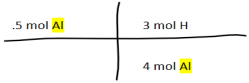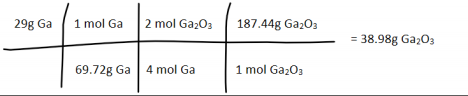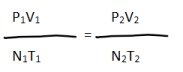×
Get Full Access to U of L - CHEM 101 - Study Guide - Midterm
Get Full Access to U of L - CHEM 101 - Study Guide - Midterm

×

U OF L / Chemistry / CHEM 101 / What refers to the sum of all atomic masses in a compound?

# What refers to the sum of all atomic masses in a compound? Description

##### Description: She posted on Blackboard that this exam will cover modules 5, 6 and 8. So that is the material in this study guide. I briefly went over module 5 as I have already covered this in previous study guides.
4 Pages 50 Views 3 Unlocks
Reviews

Chemistry Exam 3 Study Guide

## What refers to the sum of all atomic masses in a compound?∙ This exam covers modules 5, 6, and 8. I will briefly cover 5, as I  have uploaded more detailed coverage in previous study guides.  There may be previous material on the exam, but I am going off  of what she posted on Blackboard.

∙ Module 5:

∙ Molecular formula / Formula Mass: Sum off all atomic masses in  a compound.

∙ Percent composition: Divide the mass of the element by the total  mass of the compound and convert to a percent (x100). By  assuming a 100g sample, the amount in g can be converted to a  percentage. Ex: 25g of C and 75g of H can be converted to 25%  and 75% respectively.

∙ Percent composition from formula mass: Divide mass of element  by the formula mass of the total compound.

## What refers to the smallest whole number ration of elements in a compound?∙ Empirical formula: This is the smallest whole number ratio of  elements in a compound. To find this, you must divide given  amounts of element by their respective molar mass. Then divide  all products by the smallest amount received in step one. If you  get any answers like 1.5, you may need to multiply to get non decimal numbers.

∙ Empirical formula from percent composition: Assuming a 100g  sample, divide the percentage of the element by it's molar mass.  Then divide all products by the lowest amount received in step  one. You may also need to multiply here.

∙ Molecular formulas: The actual whole number ratio between  elements in a compound. Divide the molecular or molar mass by  the empirical formula to get n. Then multiply the empirical  formula by n.

## How can one form the net ionic equation?If you want to learn more check out Why do developing nations have more air pollution?
We also discuss several other topics like What does peninsula of peninsulas mean?

∙ Solutions: M= mole (solute) / L (solution). This is very self  explanatory. Just take the given amounts in the question and  plug & chug.

∙ Dilution of solution: M1V1=M2V2. You can manipulate this  formula to fit what you need to solve for. The easiest way to go  about this is to list what amounts you are given and what you  need to find. I usually make a list like M1=__, M2=__, etc. Then,  manipulating the formula, and plugging & chugging.

∙ Module 6:

∙ Balancing equations is a guess and check kind of method. Many  times you will have to find the disassociated reaction, (total)  ionic, and net ionic reactions.

∙ To disassociate an equation, you must separate the cations from  the anions. However, only aqueous (aq) compounds can be  separated. Ex: NaOH (aq), can be separated into Na, and OH  respectively. We also discuss several other topics like If you have a stronger perception of threat than efficacy what cognitive process will you experience?

∙ Writing out the equation after you have disassociated all that you  can, forms the total ionic equation.

∙ To form the net ionic equation, you take out the

elements/compounds that appear in both the reactant and  product side. The elements or compounds that you remove are  called spectator ions.

∙ Stoichiometry:

∙ A molar ratio is the ratio between elements/compounds in a  reaction. Whatever the coefficient of the element/compound, that  is the number to be used in the ratio. Ex: 4Al + 3O2 -- 4AlO So  the molar ratio would be 4 moles of Al to 3 moles of O2. (This is  just a sample reaction, I know it is not anywhere near correct.)

∙ When the question asks for moles, multiply the amount of moles  given in the equation by the molar ratio of the reaction.Don't forget about the age old question of Why do the constellations we see depend on latitude and time of year?

2

∙ Ex:∙ When the question is asking for mass, you must multiply by both  the molar mass and the molar ratio.

∙ Ex: What mass of Ga2O3 can be prepared from 29g of Ga? o 4Ga + 3O2 – 2Ga2O3 If you want to learn more check out What are pieces of music?∙ Remember, when the question asks for moles just multiply by  the given amounts by the molar ratio. When it asks you for the  mass you have to take into account the molar mass and the  molar ratio.

∙ Module 8:

∙ Pressure = force / area

∙ Units of pressure: pa – pascal, kpa – kilopascal, atm - atmosphere (760 mm Hg), tor – 1mm Hg, 1 atm = 760 tor. ∙ Pressure – P , Temperature – T , Volume – V , Number of  molecules – n.

∙ Temperature must be in Kelvin (k). Tk = Tc + 273

∙ Direct proportions:

∙ If you increase temperature, you increase pressure.

3

∙ If you increase temperature, you increase volume. ∙ If you increase pressure, you decrease volume. If you want to learn more check out What is chi square utilized for?

∙ General gas law:You can manipulate this law to conform to what you need to solve for.  Also, things that are omitted from the question can be considered  constants and can be ruled out of the equation. Many times n, is not  mentioned in the question at all so, when re-writing the equation to fit  what needs to be solved, it is left out.

4

Page ExpiredIt looks like your free minutes have expired! Lucky for you we have all the content you need, just sign up here